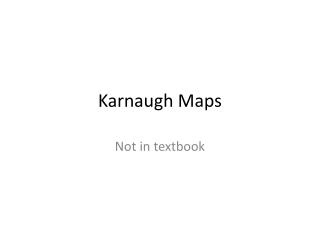DownloadDownload PresentationKarnaugh Maps

# Karnaugh Maps

Télécharger la présentation## Karnaugh Maps

- - - - - - - - - - - - - - - - - - - - - - - - - - - E N D - - - - - - - - - - - - - - - - - - - - - - - - - - -
##### Presentation Transcript

1. Karnaugh Maps Not in textbook

2. Karnaugh Maps • K-maps provide a simple approach to reducing Boolean expressions from a input-output table. • The output from the table is used to fill-in the K-map. • 1’s are used to create a Sum of Product (SOP) solution. (min terms) • 0’s are used to create a Product of Sum (POS) solution. (max terms)

3. Min Terms • Canonical representation of a Boolean expression is in the form of ^ v ~ (AND, OR, NOT). • Example: A^B v ~A^~B v A^~B (AB + AB + AB) • Candidates for canonical representation are taken from the truth table (input-output). • Candidates are identified where the output is “1”. (Max Term canonical representation candidates are identified by “0”)

4. Min Terms Min terms are taken directly from the truth tables. Where ever there is a “1” for an output, F(), we note the min term value and place a “1” in the K-map corresponding to the min term value of the table. Min term short hand is often used to replace a full input-output table. The short hand indicate the variables and the min terms that are “1”. Example: f(A,B,C) = S (1, 5, 7)

5. Examples f(A,B,C) = S (0, 1, 5, 7) f(A,B) = S (1, 2)

6. K-Map Tables • K-map tables are organized based on the number of variables. • Example: showing min terms in italic bold.

7. K-Map Examples • Reducing a Boolean expression using K-map • Identify min terms (from table or function form) • Fill-in appropriate K-map. • Group min terms in largest grouping using 4-neighbor rule. • a min term is a number if it is either to the right, left, top, or bottom. • K-map edges are connected as neighbors. • Write out the groupings as the reduced expression (circuit). f(A,B) = S (0, 3) f(A,B) = ~A^~B vA^B

8. K-Map Examples f(A,B) = S (0, 2) f(A,B) = ~B f(A,B) = S (2, 3) f(A,B) = A f(A,B) = S (0, 4) f(A,B) = BvA

9. K-Map Examples f(A,B,C) = ~A^~BvA^C f(A,B,C) = S (0, 2, 4) f(A,B,C) = ~A^~Cv~B^~C

10. K-Map Examples f(A,B,C,D) = S (5, 7, 13, 15) f(A,B,C) = B^D f(A,B,C,D) = S (0,1,2,3,8,9,10,11) f(A,B,C) =~B

11. K-Map Examples f(A,B,C,D) = S (0,1,2,8,9,10,15) f(A,B,C) = ~B^~C v~B^~D vA^B^C^D

12. HMWK Due 2/4 • Build the input-output table from the following min term list of 4-variables: S (5, 7, 10, 11, 14, 15) • Using a K-map reduce the expression from 1 such that you minimize the number of connectives (AND, OR, NOT). Remember the answer should be in the sum of product form.